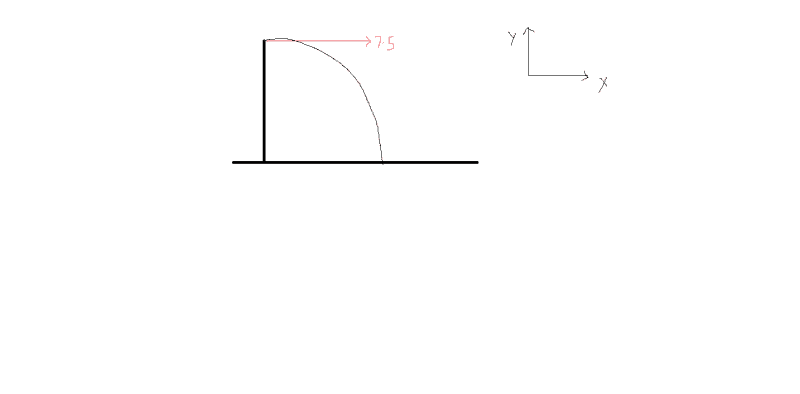# Projectile motion of a flying object

• rrosa522

## Homework Statement

an object is thrown horizontally off a cliff with an initial speed of 7.5m/s. The object strikes the ground 3 sec later
what is the horizontal velocity after 1.5 sec

## The Attempt at a Solution

i don't know how to start this problem without an angle given

## Homework Statement

an object is thrown horizontally off a cliff with an initial speed of 7.5m/s. The object strikes the ground 3 sec later
what is the horizontal velocity after 1.5 sec

## The Attempt at a Solution

i don't know how to start this problem without an angle given

What does 'horizontally' mean to you?

## Homework Statement

an object is thrown horizontally off a cliff with an initial speed of 7.5m/s. The object strikes the ground 3 sec later
what is the horizontal velocity after 1.5 sec

## The Attempt at a Solution

i don't know how to
What does 'horizontally' mean to you?
im not really sure what it is, I don't know any equations to solve this problem,

im not really sure what it is, I don't know any equations to solve this problem,
Well, if you have a dictionary you could look up the definition of 'horizontally'. You can probably find some dictionaries online if you are diligent.

(Hint: 'horizontally' and 'vertically' are often used in these types of projectile problems. You should become familiar with their definitions.)

Well, if you have a dictionary you could look up the definition of 'horizontally'. You can probably find some dictionaries online if you are diligent.

(Hint: 'horizontally' and 'vertically' are often used in these types of projectile problems. You should become familiar with their definitions.)
i think it is the same as the initial speed given so 7.5m/s, is that right?

i think it is the same as the initial speed given so 7.5m/s, is that right?

The initial speed is the initial speed, no more, no less.

You were originally looking for the initial angle at which the projectile was thrown. Knowing the definition of 'horizontally' provides that angle, no more, no less.

Hi rosa. A ball is thrown horizontally--- this means the ball is thrown at an angle of 0 deg. with the X-axis.What are the forces acting on the ball in X-direction (or horizontal direction)? What is the acceleration of the ball in horizontal direction?